关于C语言下的atof的小问题

```int main(int argc, char **argv) {
char str_int = "435";
char str_float = "432.44";
char *s = "45.344 soft";

int num_int = atoi(str_int);
float num_float = atof(str_float);

printf("num_int:%d. num_float:%f. test:%d. s:%f\n", num_int, num_float, atof("abcd"), atof(s));
}```
gcc foo.c -o foo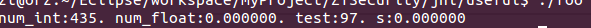0
j

double atof(const char *);

int main(int argc, char **argv) {

char str_int = "435";
char str_float = "432.44";
char *s = "45.344 soft";

int num_int = atoi(str_int);
float num_float = atof(str_float);
printf("num_int:%d. num_float:%f. test:%d. s:%f\n", num_int, num_float, atof("abcd"), atof(s));
}

0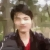```tsl0922s-air:~ tsl0922\$ gcc foo.c -o foo
foo.c:12:81: warning: format specifies type 'int' but the argument has type
'double' [-Wformat]
...num_float:%f. test:%d. s:%f\n", num_int, num_float, atof("abcd"), atof(s));
~~                               ^~~~~~~~~~~~
%f
1 warning generated.
tsl0922s-air:~ tsl0922\$ ./foo
num_int:435. num_float:432.440002. test:5. s:0.000000```0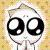printf("num_int:%d. num_float:%f. test:%d. s:%f\n", num_int, num_float,atoi("abcd"), (float)atof(s));

0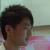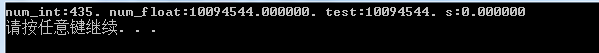0```tsl0922s-air:~ tsl0922\$ gcc foo.c -o foo
tsl0922s-air:~ tsl0922\$ ./foo
num_int:435. num_float:432.440002. test:0.000000. s:45.344000```

```#include <stdio.h>
#include <stdlib.h>

int main(int argc, char **argv) {
char str_int = "435";
char str_float = "432.44";
char *s = "45.344 soft";

int num_int = atoi(str_int);
float num_float = atof(str_float);

printf("num_int:%d. num_float:%f. test:%f. s:%f\n", num_int, num_float, atof("abcd"), atof(s));
}```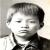00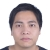printf("num_int:%d num_float:%f test:%d s:%f  \r\n", num_int, num_float, (int)(atof("abcd")), atof(s));

0

So, 有时候不关注别人的警告的话，你有可能会栽跟头。

（补：既然有些朋友不认同我这个答案，希望你们能够继续研究一下，争取把这个坑给彻底填平了，我精神上非常支持你们的做法，但是我没有时间参与一起研究，抱歉。今晚，我会来这里“收割”一下答案，如果有好的答案，我会改正。0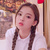```sscanf(str_int, "%d", &num_int);
sscanf(str_float, "%f", &num_float);```